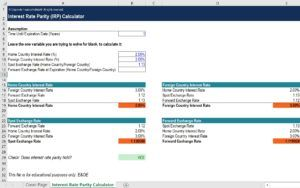# Interest Rate Parity (IRP) Calculator

## Interest Rate Parity (IRP) Excel Calculator

This interest rate parity (IRP) calculator can be used to calculate any of the components in the IRP equation. IRP shows the relationship between the exchange rate and interest rates of two countries. In order for currencies to be in equilibrium, this parity should hold.

Below is a quick preview of CFI’s interest rate parity (IRP) calculator:To download CFI’s free calculator, check out the CFI Marketplace: Interest Rate Parity (IRP) Calculator

### About the Interest Rate Parity (IRP)

The interest rate parity demonstrates the relationship between interest rates and the exchange rate of two countries. Decisions to alter interest rates can lead to the appreciation or depreciation of currencies relative to foreign currencies. This relationship also shows that if parity were to be held then an investor should not be able to profit solely from borrowing money and investing in another currency. IRP is important to consider in the pricing of exchange rate forwards and can be used to identify potential mispricing.

If this parity relationship is not being held then this can give rise to a potential arbitrage opportunity. For example, if you were to calculate the forward exchange rate based on IRP, but the actual forward rate was higher then this could be a potential opportunity to profit. You could borrow money, exchange it at the spot rate, invest in the foreign currency, and buy a forward contract. At the expiration date, you could exchange your money back to your home currency and pay back the loan. You would be left with a profit made with purely borrowed funds.

This calculator involves all the components of the IRP equation. The first component is the time until expiration you set yourself based on the forward contract you are considering. The other four variables you can change in this calculator are the following:

• Spot Exchange Rate
• Forward Exchange Rate
• Home Interest Rate
• Foreign Interest Rate

### Financial Analyst Certification

Become a certified Financial Modeling and Valuation Analyst (FMVA)® by completing CFI’s online financial modeling classes and training program!